Math Calculators, Lessons and Formulas

It is time to solve your math problem

mathportal.org
• Geometry
• Cone

# Cone

ans:
syntax error
C
DEL
ANS
±
(
)
÷
×
7
8
9
4
5
6
+
1
2
3
=
0
.
auto next question
calculator
•  Question 1: 1 pts The net of a cone consists of the following two parts: a circle that gives the base and a sector that gives the curved surface.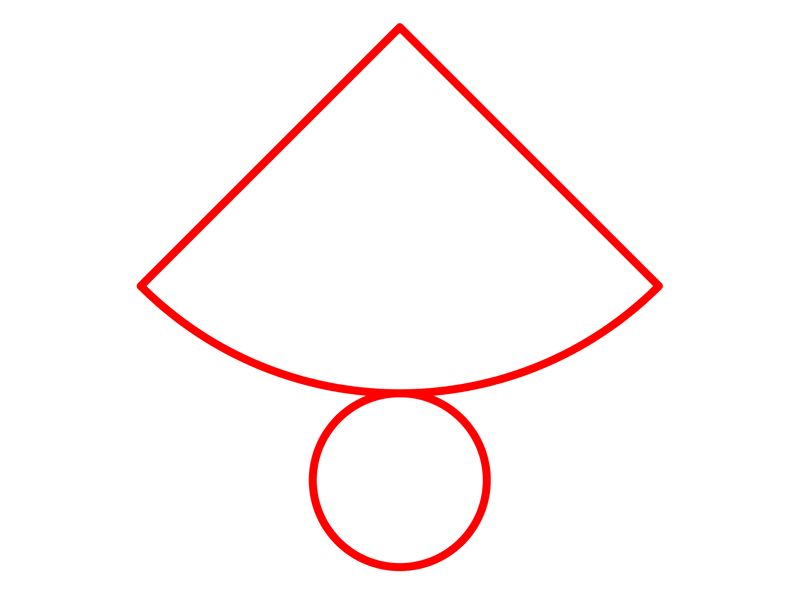•  Question 2: 1 pts We can see on this picture the cone and its net.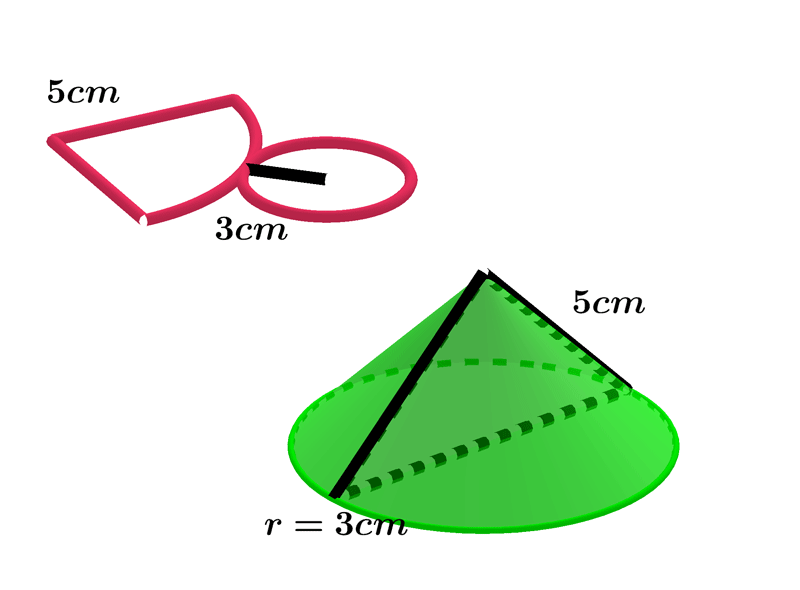•  Question 3: 1 pts Find the volume of the cone shown on the picture?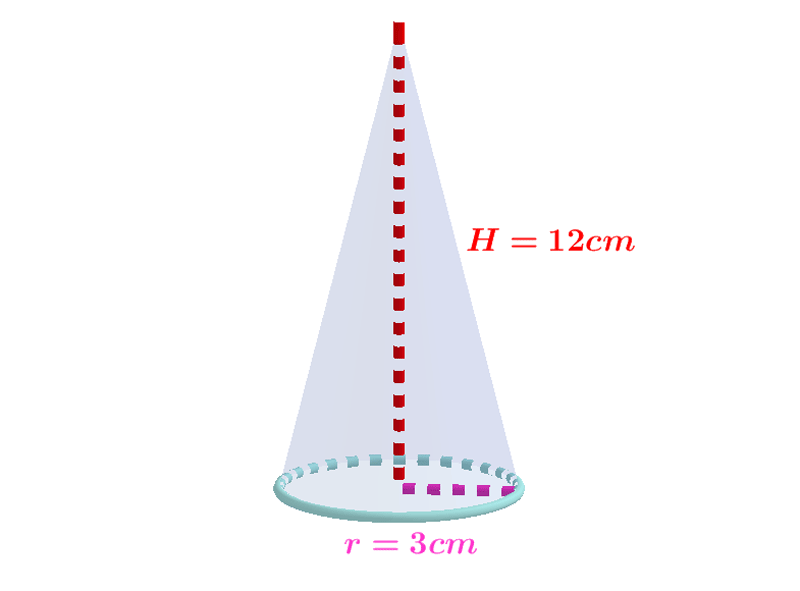$V=27\pi cm^{3}$ $V=36\pi cm^{3}$ $V=63\pi cm^{3}$
•  Question 4: 1 pts Find the surface area of the cone shown on the picture.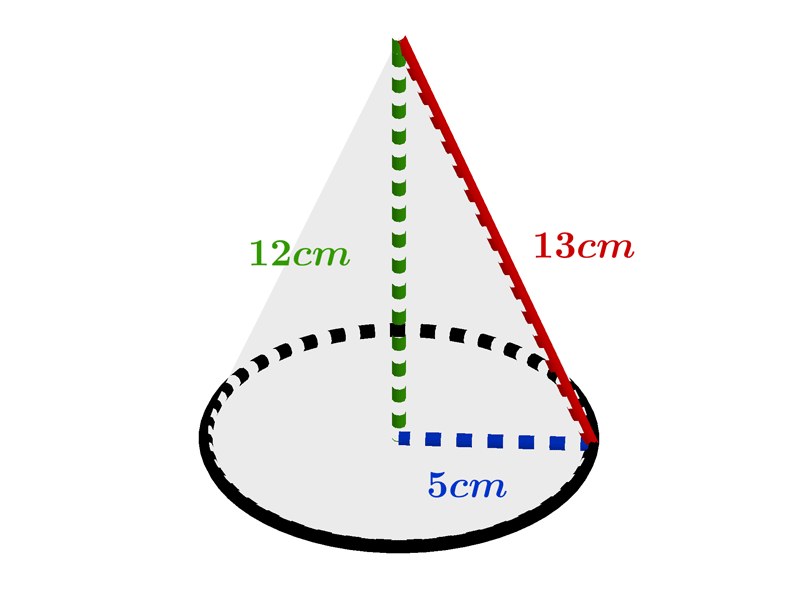Area $=$
•  Question 5: 2 pts Find the volume of the cone shown on the picture?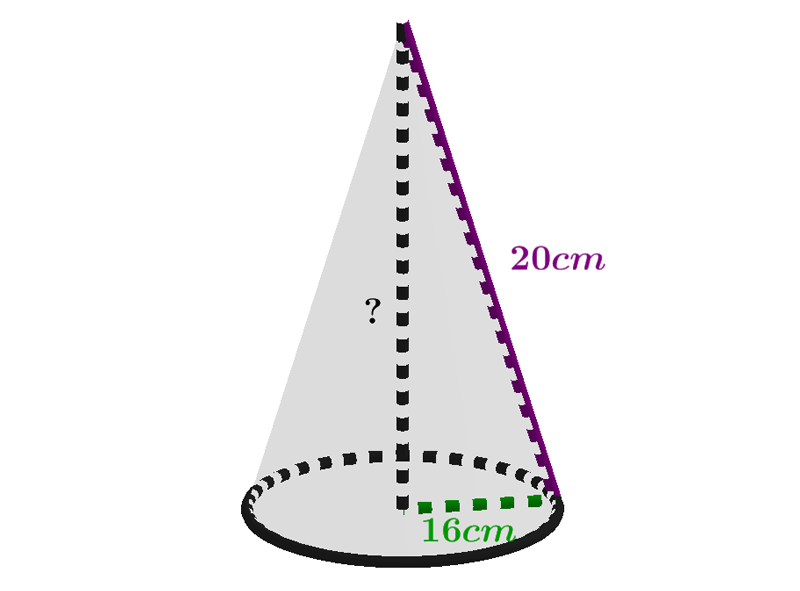$V=1204\pi cm^{3}$ $V=1024\pi cm^{3}$ $V=968\pi cm^{3}$ $V=1032\pi cm^{3}$
•  Question 6: 2 pts What is formula of finding volume of frustum of a cone?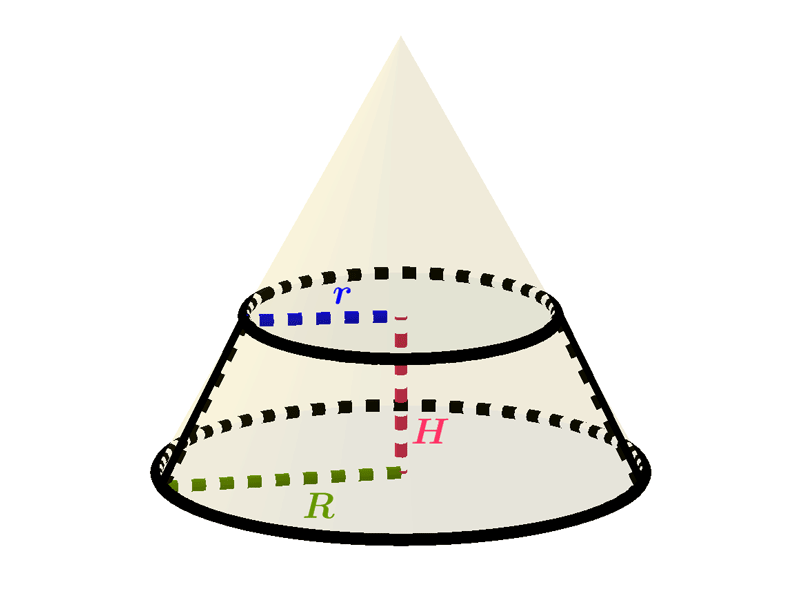$V=\dfrac{\pi H}{3}\left(R^{2}+Rr+r^{2}\right)$ $V=\dfrac{\pi}{3}\left(R^{2}+Rr+r^{2}\right)$ $V=\dfrac{\pi H}{3}\left(R^{2}+\sqrt{Rr}+r^{2}\right)$ $V=\dfrac{\pi H}{3}\left(R^{2}+R^{2}r^{2}+r^{2}\right)$
•  Question 7: 1 pts The lateral surface area of a cone base with radius $r$ and height $H$ is $$\pi r \sqrt{r^{2}+H^{2}}.$$
•  Question 8: 3 pts The total surface area of a cone is $24\pi cm ^{2}$, and the area of its base is $9\pi cm ^{3}.$ Find the volume of that cone.
 $V=24\pi cm^{3}$ $V=36\pi cm^{3}$ $V=12\pi cm^{3}$ $V=72\pi cm^{3}$
•  Question 9: 3 pts The volume of the frustum is $584\pi cm^{3}$, and the radii of its circular ends are $10 cm$ and $7 cm.$ Find the height of that frustum.
 $8cm$ $10cm$ $12cm$ $16cm$
•  Question 10: 3 pts A right circular cone is the cone in which the altitude or height is exactly perpendicular to the Radius or the circle. Whereas a cone is a 3D figure with one surface curved and a base surface as a circle. A cone may be right circular or may not.
•  Question 11: 3 pts Find the ratio of volumes of right circular cone and square pyramid shown on the picture?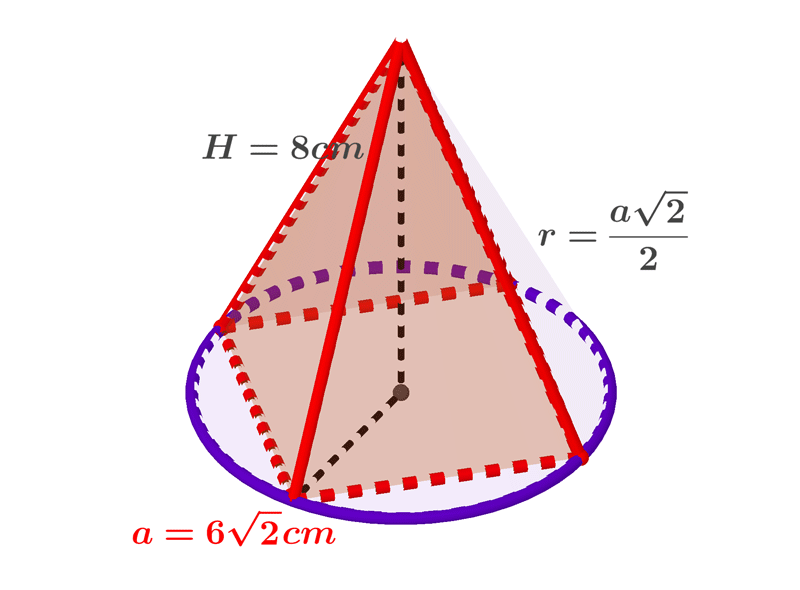V_{c}:V_{p}=$•  Question 12: 3 pts Find the volume of the solid shown on the picture.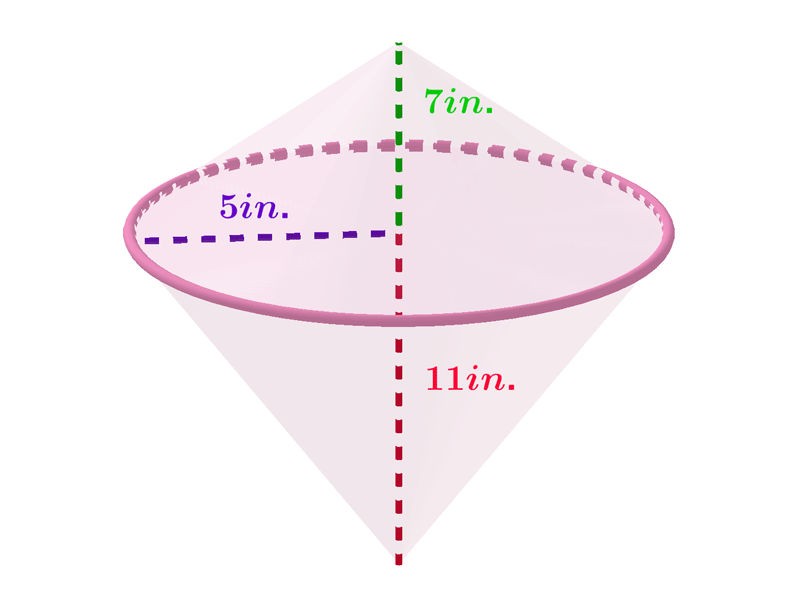$V=210\pi cm^{3}V=180\pi cm^{3}V=150\pi cm^{3}\$